# Resources tagged with: Addition & subtraction

Filter by: Content type:
Age range:
Challenge level:

### There are 73 results

Broad Topics > Calculations and Numerical Methods > Addition & subtraction### Summing Consecutive Numbers

##### Age 11 to 14Challenge Level

15 = 7 + 8 and 10 = 1 + 2 + 3 + 4. Can you say which numbers can be expressed as the sum of two or more consecutive integers?### Magic Letters

##### Age 11 to 14Challenge Level

Charlie has made a Magic V. Can you use his example to make some more? And how about Magic Ls, Ns and Ws?### Consecutive Negative Numbers

##### Age 11 to 14Challenge Level

Do you notice anything about the solutions when you add and/or subtract consecutive negative numbers?### Multiply the Addition Square

##### Age 11 to 14Challenge Level

If you take a three by three square on a 1-10 addition square and multiply the diagonally opposite numbers together, what is the difference between these products. Why?### Number Pyramids

##### Age 11 to 14Challenge Level

Try entering different sets of numbers in the number pyramids. How does the total at the top change?### Aba

##### Age 11 to 14Challenge Level

In the following sum the letters A, B, C, D, E and F stand for six distinct digits. Find all the ways of replacing the letters with digits so that the arithmetic is correct.### Got it for Two

##### Age 7 to 14Challenge Level

Got It game for an adult and child. How can you play so that you know you will always win?### Magic Squares for Special Occasions

##### Age 11 to 16

This article explains how to make your own magic square to mark a special occasion with the special date of your choice on the top line.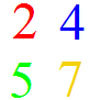### And So on and So On

##### Age 11 to 14Challenge Level

If you wrote all the possible four digit numbers made by using each of the digits 2, 4, 5, 7 once, what would they add up to?### Making a Difference

##### Age 11 to 14Challenge Level

How many different differences can you make?### Pole Star Sudoku 2

##### Age 11 to 16Challenge Level

This Sudoku, based on differences. Using the one clue number can you find the solution?### Eleven

##### Age 11 to 14Challenge Level

Replace each letter with a digit to make this addition correct.### Two and Two

##### Age 11 to 16Challenge Level

How many solutions can you find to this sum? Each of the different letters stands for a different number.### Crossed Ends

##### Age 11 to 14Challenge Level

Crosses can be drawn on number grids of various sizes. What do you notice when you add opposite ends?### Clocked

##### Age 11 to 14Challenge Level

Is it possible to rearrange the numbers 1,2......12 around a clock face in such a way that every two numbers in adjacent positions differ by any of 3, 4 or 5 hours?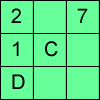### Alphabetti Sudoku

##### Age 11 to 16Challenge Level

This Sudoku requires you to do some working backwards before working forwards.### Charitable Pennies

##### Age 7 to 14Challenge Level

Investigate the different ways that fifteen schools could have given money in a charity fundraiser.### Cunning Card Trick

##### Age 11 to 14Challenge Level

Delight your friends with this cunning trick! Can you explain how it works?### Have You Got It?

##### Age 11 to 14Challenge Level

Can you explain the strategy for winning this game with any target?### Postage

##### Age 14 to 16Challenge Level

The country Sixtania prints postage stamps with only three values 6 lucres, 10 lucres and 15 lucres (where the currency is in lucres).Which values cannot be made up with combinations of these postage. . . .### Number Daisy

##### Age 11 to 14Challenge Level

Can you find six numbers to go in the Daisy from which you can make all the numbers from 1 to a number bigger than 25?### Double Digit

##### Age 11 to 14Challenge Level

Choose two digits and arrange them to make two double-digit numbers. Now add your double-digit numbers. Now add your single digit numbers. Divide your double-digit answer by your single-digit answer. . . .### Up, Down, Flying Around

##### Age 11 to 14Challenge Level

Play this game to learn about adding and subtracting positive and negative numbers### Adding All Nine

##### Age 11 to 14Challenge Level

Make a set of numbers that use all the digits from 1 to 9, once and once only. Add them up. The result is divisible by 9. Add each of the digits in the new number. What is their sum? Now try some. . . .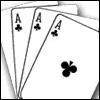### Amazing Card Trick

##### Age 11 to 14Challenge Level

How is it possible to predict the card?### Got it Article

##### Age 7 to 14

This article gives you a few ideas for understanding the Got It! game and how you might find a winning strategy.### Weights

##### Age 11 to 14Challenge Level

Different combinations of the weights available allow you to make different totals. Which totals can you make?### Diagonal Sums

##### Age 7 to 14Challenge Level

In this 100 square, look at the green square which contains the numbers 2, 3, 12 and 13. What is the sum of the numbers that are diagonally opposite each other? What do you notice?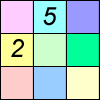### Difference Sudoku

##### Age 14 to 16Challenge Level

Use the differences to find the solution to this Sudoku.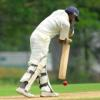### Twenty20

##### Age 7 to 16Challenge Level

Fancy a game of cricket? Here is a mathematical version you can play indoors without breaking any windows.### Making Maths: Double-sided Magic Square

##### Age 7 to 14Challenge Level

Make your own double-sided magic square. But can you complete both sides once you've made the pieces?### Cinema Problem

##### Age 11 to 14Challenge Level

A cinema has 100 seats. Show how it is possible to sell exactly 100 tickets and take exactly Â£100 if the prices are Â£10 for adults, 50p for pensioners and 10p for children.### Countdown Fractions

##### Age 11 to 16Challenge Level

Here is a chance to play a fractions version of the classic Countdown Game.### Calendar Capers

##### Age 11 to 14Challenge Level

Choose any three by three square of dates on a calendar page...### Largest Number

##### Age 11 to 14Challenge Level

What is the largest number you can make using the three digits 2, 3 and 4 in any way you like, using any operations you like? You can only use each digit once.### Arrange the Digits

##### Age 11 to 14Challenge Level

Can you arrange the digits 1,2,3,4,5,6,7,8,9 into three 3-digit numbers such that their total is close to 1500?### Pairs

##### Age 11 to 14Challenge Level

Ann thought of 5 numbers and told Bob all the sums that could be made by adding the numbers in pairs. The list of sums is 6, 7, 8, 8, 9, 9, 10,10, 11, 12. Help Bob to find out which numbers Ann was. . . .### Subtraction Surprise

##### Age 7 to 14Challenge Level

Try out some calculations. Are you surprised by the results?### An Introduction to Magic Squares

##### Age 7 to 16

Find out about Magic Squares in this article written for students. Why are they magic?!### Score

##### Age 11 to 14Challenge Level

There are exactly 3 ways to add 4 odd numbers to get 10. Find all the ways of adding 8 odd numbers to get 20. To be sure of getting all the solutions you will need to be systematic. What about. . . .### Forwards Add Backwards

##### Age 11 to 14Challenge Level

What happens when you add a three digit number to its reverse?### Cubes Within Cubes

##### Age 7 to 14Challenge Level

We start with one yellow cube and build around it to make a 3x3x3 cube with red cubes. Then we build around that red cube with blue cubes and so on. How many cubes of each colour have we used?### As Easy as 1,2,3

##### Age 11 to 14Challenge Level

When I type a sequence of letters my calculator gives the product of all the numbers in the corresponding memories. What numbers should I store so that when I type 'ONE' it returns 1, and when I type. . . .### Cayley

##### Age 11 to 14Challenge Level

The letters in the following addition sum represent the digits 1 ... 9. If A=3 and D=2, what number is represented by "CAYLEY"?### Football Sum

##### Age 11 to 14Challenge Level

Find the values of the nine letters in the sum: FOOT + BALL = GAME### Digit Sum

##### Age 14 to 16 ShortChallenge Level

What is the sum of all the digits in all the integers from one to one million?### 3388

##### Age 11 to 14Challenge Level

Using some or all of the operations of addition, subtraction, multiplication and division and using the digits 3, 3, 8 and 8 each once and only once make an expression equal to 24.### Tis Unique

##### Age 11 to 14Challenge Level

This addition sum uses all ten digits 0, 1, 2...9 exactly once. Find the sum and show that the one you give is the only possibility.### Add to 200

##### Age 11 to 14Challenge Level

By selecting digits for an addition grid, what targets can you make?### Consecutive Numbers

##### Age 7 to 14Challenge Level

An investigation involving adding and subtracting sets of consecutive numbers. Lots to find out, lots to explore.